## Example Questions

### Example Question #1 : How To Find The Perimeter Of A Quadrilateral

A homeowner wants to set up a rectangular enclosure for his dog. The plot of land that the enclosure will enclose measures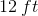by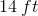. What is the length in feet of chain link fence the owner will need to create a fence around the enclosure?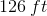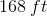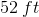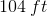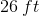Explanation:

To answer this question, we must find the perimeter of the fence the homeowner is wanting to create.

To find the perimeter of a rectangle, we multiply the length by two, multiply the width by two, and add these two numbers together. The equation can be represented as this: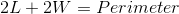We must then plug in our values ofandgiven to us for the length and width.

So for this data: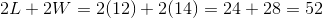Therefore, the amount of fencing needed to fully surround the dog's enclosure is.